# Bianchi surface

(diff) ← Older revision | Latest revision (diff) | Newer revision → (diff)

A surface of negative Gaussian curvaturewhich can be expressed in asymptotic parameters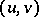as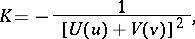where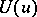and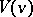are arbitrary functions; thus, Bianchi surfaces can be characterized by the fact that the function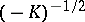is diagonal with respect to its asymptotic net, i.e.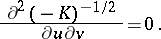For instance, a ruled Bianchi surface is a conoid — a surface attached to the Peterson surface. If a Bianchi surface is given, it is possible to determine the classes of surfaces which can be obtained by a deformation over a principal base and to classify them. Thus, if the principal base contains two families of geodesic lines, the functions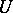andare constant and the bent surface is a Voss surface (the class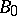).

The class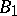is characterized by the fact that only one family of lines of the principal base are geodesics (one of the functions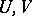is constant); conoids may serve as an example. The classcorresponds to functionswhich both depend non-trivially on their arguments. See also Bianchi congruence.